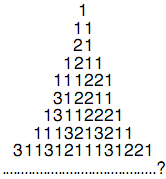# What is the Pattern Puzzle - Solution

## The Puzzle:

Write down the next line in the following pattern:## Our Solution:

Each row describes the grouping of the digits in the line above.

The top row "1" has one one, so we write "11" for the second row.
The second row "11" has two ones, so we write "21" for the third row.
And so on.

To describe the line: 31131211131221
We say: one three, two ones, one three, one one, one two, three ones, one three, one one, two twos and one one.

Which is: 13211311123113112211
See this puzzle without solution
Discuss this puzzle at the Math is Fun Forum